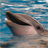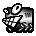## How to read Status of INPUT GPIO pin?

cancel
Showing results for
Did you mean:

## How to read Status of INPUT GPIO pin?

2,039 ViewsContributor I

I am using FRDM-KE04Z dev board with onboard MKE04Z8VFK4 MCU, for developing Bit banging I am able to transmit data through gpio pin but I am unable to receive data from gpio pin, I need to know how to read the status of input GPIO pin?

below is my sample code: I am configured PTA3 is OUTPUT gpio and PTA2 Input gpio pin

{
unsigned char DataValue = 0;
uint32_t data;

while((GPIO_PinRead(kGPIO_PORTA, 2U)) == 0);  // this is the right way?

PIT_SetTimerPeriod(PIT, kPIT_Chnl_0, USEC_TO_COUNT(OneBitDelay, PIT_SOURCE_CLOCK));
PIT_StartTimer(PIT, kPIT_Chnl_0);
while(!pitIsrFlag);
PIT_StopTimer(PIT, kPIT_Chnl_0);
pitIsrFlag = 0;

PIT_SetTimerPeriod(PIT, kPIT_Chnl_0, USEC_TO_COUNT((OneBitDelay/2), PIT_SOURCE_CLOCK));
PIT_StartTimer(PIT, kPIT_Chnl_0);
while(!pitIsrFlag);
PIT_StopTimer(PIT, kPIT_Chnl_0);
pitIsrFlag = 0;

PIT_SetTimerPeriod(PIT, kPIT_Chnl_0, USEC_TO_COUNT(OneBitDelay, PIT_SOURCE_CLOCK));

for ( unsigned char i = 0; i < DataBitCount; i++ )
{
if ( (GPIO_PinRead(kGPIO_PORTA, 2U)))  // this is the right way?
{
DataValue += (1<<i);
}

//DelayUs(OneBitDelay);
PIT_StartTimer(PIT, kPIT_Chnl_0);
while(!pitIsrFlag);
PIT_StopTimer(PIT, kPIT_Chnl_0);
pitIsrFlag = 0;
}

// Check for stop bit
if ( (GPIO_PinRead(kGPIO_PORTA, 2U)))  // this is the right way?
{

PIT_SetTimerPeriod(PIT, kPIT_Chnl_0, USEC_TO_COUNT((OneBitDelay/2), PIT_SOURCE_CLOCK));
PIT_StartTimer(PIT, kPIT_Chnl_0);
while(!pitIsrFlag);
PIT_StopTimer(PIT, kPIT_Chnl_0);
pitIsrFlag = 0;

return DataValue;
}
else //some error occurred !
{
PIT_SetTimerPeriod(PIT, kPIT_Chnl_0, USEC_TO_COUNT((OneBitDelay/2), PIT_SOURCE_CLOCK));
PIT_StartTimer(PIT, kPIT_Chnl_0);
while(!pitIsrFlag);
PIT_StopTimer(PIT, kPIT_Chnl_0);
pitIsrFlag = 0;
//DelayUs(OneBitDelay/2);
return 0x000;
}
}

void UART_Transmit(const char DataValue)
{
/* Basic Logic

TX pin is usually high. A high to low bit is the starting bit and
a low to high bit is the ending bit. No parity bit. No flow control.
BitCount is the number of bits to transmit. Data is transmitted LSB first.

*/

// Send Start Bit
//GPIO_PinClear(UART_TX);
GPIO_PinWrite(kGPIO_PORTA, 3U, 0); ////////////////////////////////////

//DelayUs(OneBitDelay);
PIT_StopTimer(PIT, kPIT_Chnl_0);
PIT_SetTimerPeriod(PIT, kPIT_Chnl_0, USEC_TO_COUNT(OneBitDelay, PIT_SOURCE_CLOCK));
PIT_StartTimer(PIT, kPIT_Chnl_0);
while(!pitIsrFlag);
PIT_StopTimer(PIT, kPIT_Chnl_0);
pitIsrFlag = 0;

PIT_SetTimerPeriod(PIT, kPIT_Chnl_0, USEC_TO_COUNT(OneBitDelay, PIT_SOURCE_CLOCK));

for ( unsigned char i = 0; i < DataBitCount; i++ )
{
//Set Data pin according to the DataValue
if( ((DataValue>>i)&0x1) == 0x1 ) //if Bit is high
{
//GPIO_PinSet(UART_TX);
GPIO_PinWrite(kGPIO_PORTA, 3U, 1); ////////////////////////////////////
}
else //if Bit is low
{
//GPIO_PinClear(UART_TX);
GPIO_PinWrite(kGPIO_PORTA, 3U, 0); ////////////////////////////////////
}
PIT_StartTimer(PIT, kPIT_Chnl_0);
while(!pitIsrFlag);
PIT_StopTimer(PIT, kPIT_Chnl_0);
pitIsrFlag = 0;
//DelayUs(OneBitDelay);
}

//Send Stop Bit
//GPIO_PinSet(UART_TX);
GPIO_PinWrite(kGPIO_PORTA, 3U, 1); ////////////////////////////////////

//DelayUs(OneBitDelay);
PIT_SetTimerPeriod(PIT, kPIT_Chnl_0, USEC_TO_COUNT(OneBitDelay, PIT_SOURCE_CLOCK));
PIT_StartTimer(PIT, kPIT_Chnl_0);
while(!pitIsrFlag);
PIT_StopTimer(PIT, kPIT_Chnl_0);
pitIsrFlag = 0;
}

Labels (9)

• ### USB

2 Replies
883 ViewsNXP TechSupport

Hi, Vikranth,

If you want to configure the PTA2 as input, I think you can use the code:

gpio_pin_config_t input_config = {
kGPIO_DigitalInput, 0,
};

/* Init input GPIO. */
GPIO_PinInit(kGPIO_PORTA, 2, &input_config);

GPIO_PinRead(kGPIO_PORTA,2); //If PTA2 is high logic, it returns 0x01, if vPTA2 is low logic, it will return 0

Pls have a try

Regarding your code, it seems that you read the uart receiver logic, I suggest you use uart module instead of sampling the logic of uart_rx pin.

BR

Xiangjun Rong

This is the api function in ksdk

{
uint8_t instance = (uint8_t)port / PORT_NUMBERS_EACH_GPIO;
uint8_t shift = (uint8_t)port % PORT_NUMBERS_EACH_GPIO;
GPIO_Type *base = s_gpioBases[instance];

return (((base->PDIR) >> ((uint32_t)pin + (shift * PIN_NUMBERS_EACH_PORT))) & 0x01U);
}

883 ViewsSpecialist V

Hi

I don't know the routine

but if it doesn't work as it is (and you have referenced the correct port and bit - PTA2) it may be that it needs to be used as follows instead: